# Karl Schwarzschild facts for kids

Kids Encyclopedia Facts
Karl Schwarzschild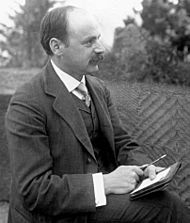Karl Schwarzschild (1873–1916)
Born 9 October 1873
Died 11 May 1916 (aged 42)
Potsdam, German Empire
Nationality German
Alma mater Ludwig Maximilian University of Munich
Spouse(s) Else Rosenbach
Scientific career
Fields Physics
Astronomy
Influenced Martin Schwarzschild
Signature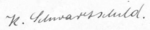Karl Schwarzschild (9 October 1873 – 11 May 1916) was a German physicist and astronomer.

Best known for his solution to Einstein's equations of general relativity, which describes a the gravitational field outside a non-rotating black hole with no magnetic field.

The equation describes how a particle moves space near this black hole (using spherical coordinates).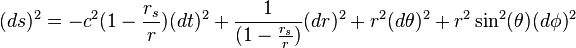$(ds)^2=-c^2(1-\frac{r_s}{r})(dt)^2+\frac{1}{(1-\frac{r_s}{r})}(dr)^2+r^2(d\theta)^2+r^2\sin^2(\theta)(d\phi)^2$

ds is the path it follows
dt is the change in time
dr is the change in radius
d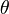$\theta$ and d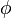$\phi$ are the change in angle$\theta$ is also the angle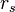$r_s$ is the set radius limit of the heavy object
r is how close the travelling object is to the heavy object
c is the speed of light

This equation is also known as the Schwarzschild metric or Schwarzschild solution.

## Images for kidsKarl Schwarzschild Facts for Kids. Kiddle Encyclopedia.